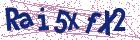# Regularization of the generalized auto-convolution Volterra integral equation of the first kind

Document Type : Research Article

Authors

Department of Mathematics, Faculty of Sciences, Urmia University, Urmia, Iran.

Abstract

In this paper,  a generalized version of the auto-convolution Volterra integral equation of the first kind as an ill-posed problem is studied. We apply the piecewise polynomial collocation method to reduce the numerical solution of this equation to a system of algebraic equations. According to the proposed numerical method, for $n=0$ and  $n=1,\ldots, N-1$, we obtain a  nonlinear and linear system, respectively. We have to distinguish between two cases, nonlinear and linear systems of algebraic equations. A double iteration process based on the modified Tikhonov regularization method is considered to solve the nonlinear algebraic equations. In this process, the outer iteration controls the evolution path of the unknown vector $U_0^{\delta}$ in the selected direction $\tilde{u}_0$, which is determined from the inner iteration process. For the linear case, we apply the Lavrentiev $\tilde{m}$ times iterated regularization method to deal with the ill-posed linear system. The validity and efficiency of the proposed method are demonstrated by several numerical experiments.

Keywords20.1001.1.24236977.2023.13.2.4.7

Main Subjects

#### References

 Brunner, H. Volterra integral equations: An introduction to theory and applications, Cambridge University Press, Cambridge/UK, 2017.
 Choi, K. and Lanterman, A.D. An iterative deautoconvolution algorithm for nonnegative functions, Inverse Prob., 21 (2005) 981–995.
 Dai, Z. and Lamm P.K. Local regularization for the nonlinear inverse autoconvolution problem, SIAM J. Numer. Anal., 46 (2008) 832–868.
 Feng, L. and Yang, X. Spectral regularization method for Volterra integral equation of the first kind with noise data, Acta Math. Sci. Ser. A, 40 (3)(2020) 650–661.
 Fleischer, G. and Hofmann, B. On inversion rates for the auto convolu-tion equation, Inverse Prob. 12 (1996) 419–435.
 Fleischer, G. and Hofmann, B. The local degree of ill-posedness and the autoconvolution equation, Nonlinear Anal. 30 (1997) 3323–3332.
 Fleischer, G., Gorenflo, R. and Hofmann, B. On the autoconvolution equation and total variation constraints, Z. Angew. Math. Mech., 79(1999) 149–159.
 Gerth, D., Hofman, B., Birkholz, S. and Koke, S.Regularization of an au-toconvolution problem in ultrashort laser pulse characterization, Inverse Probl. Sci. Eng. 22(2) ( 2013) 245–266.
 Gorenflo, R. and Hofmann, B. On autoconvolution and regularization, Inverse Prob., 10 (1994) 353–373.
 Janno, J. On a regularization method for the autoconvolution equation, Z. Angew. Math. Mech., 77 (1997) 393–394.
 Janno, J. Lavrent’ev regularization of ill-posed problems containing non-linear near-to-monotone operators with application to auto-convolution equation, Inverse Prob., 16 (2000) 333–348.
 Jozi, M. and Karimi, S. Direct implementation of Tikhonov regular-ization for the first kind integral equation J. Comp. Math., 40, (2022)335–353.
 Karakeev, T.T. and Imanaliev T. M. Regularization of Volterra linear integral equations of the first kind with the smooth data, Lobachevskii J. Math., 41 (2020) 39–45.
 Lamm, P.K. A survey of regularization methods for first-kind Volterra equations. Colton D., Engl H. W., Louis H. K., McLaughlin J. R., Run-dell W, eds. Surveys on Solution Methods for Inverse Problems. Vienna: Springer, (2000) 53–82.
 Liu, C.S. Optimally generalized regularization methods for solving linear inverse problems, CMC: Computers, Materials, Continua, vol. 29 (2012)103–127.
 Masouri, Z. and Hatamzadeh, S. A regularization-direct method to nu-merically solve first kind Fredholm integral equation, KYUNGPOOK Math. J. 60 (2020) 869–881.
 Plato, R. Iterative and parametric methods for linear ill-posed problems, TU Berlin, 1995.
 Ramlau, R. Morozov’s discrepancy principle for Tikhonov-regularization of nonlinear operators, Numer. Funct. Anal. Optim., 23 (2002) 147–172.
 Richter, M. Approximation of Gussian Random Element and Statistics, Stuttgart, B.G. Teubner, 1992.
 Titchmarsh, E.C. The zeros of certain integral functions, Proc. London Math. Society. 25 (1926) 283–302.
 Weichung, Y., Chan I-Yao, Cheng-Yu Ku,Chia-Ming Fan, Pai-Chen G A double iteration process for solving the nonlinear algebraic equations, especially for ill-posed nonlinear algebraic equations, J. Comput. Model. Eng. Sci. 99(2) (2014) 123–149.
 Wolfersdorf, L.V. Autoconvolution equations and special functions, In-tegral Transforms Spec. Funct., 19 (2008) 677–686.
 Wolfersdorf, L.V. Einige Klassen quadratischer Integralgleichungen [Some classes of quadratic integral equations], Sitz.ber. Sachs. Akad. Wiss. Leipz. Math.-Nat.wiss. Kl. 2000.
 Wolfersdorf, L.V. Auto convolution equations and special functions II, Integral Transforms Spec. Funct., 21(4) (2010) 295–306.
 Ziyaee, F. and Tari, A. Regularization method for the two-dimensional Fredholm integral equations of the first kind, Int. J. Nonlinear Sci., 18(2014) 189–194.
 Zhang, Y., Forssen, P., Fornstedt, T., Gulliksson M. and Dai X. An adap-tive regularization algorithm for recovering the rate constant distribution from biosensor data, Inverse Probl. Sci. Eng., 26 (2018) 1464–1489.
 Zhang, R., Liang, H. and Brunner, H. Analysis of collocation methods for generalized autoconvolution Volterra integral equations, SIAM J. Numer. Anal., Vol. 54 (2) (2016) 899–920.
 Zhang, Y., Lukyanenko, D. and Yagola, A. Using Lagrange principle for solving two-dimensional integral equation with a positive kernel, Inverse Probl. Sci. Eng., 24(5) (2016) 811–831.
 Zhang, Y., Lukyanenko, D. and Yagola, A. An optimal regularization method for convolution equations on the sourcewise represented set, J Inverse Ill-Pose P., 23(5) (2016) 465–475.
 Zhang. Y., Gulliksson, M., Hernandez, B.V. and Schaffernicht, E. Re-constructing gas distribution maps via an adaptive sparse regularization algorithm, Inverse Probl. Sci. Eng,. 24(7) (2016) 1186–1204.